# On the Maksa-Volkmann functional inequality |f(x+y)|≥|f(x)+f(y)| when the range of f is a space of functions

### Abstractcarpathian_2014_30_2_253_256_abstract

P. Volkmann functional inequality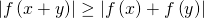is extended to functions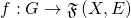where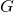is an
additive group and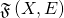is the space of functions from a set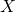to a linear normed space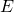. As a corollary one proves that an operator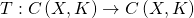which satisfies the functional inequality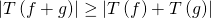,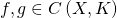is additive. Here we denoted bya compact topological space,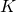is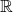or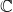and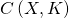is the linear space of continuous functions defined onwith values in.